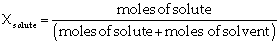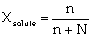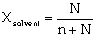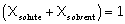# Chemistry Assignment Help With Mole Fraction (X)

## Mole Fraction (X)

A simple solution is made of two substances; one is the solute and the other is solvent. Mole fraction, X, of solute is defined as the ratio of the number of moles of solute and the total number of moles of solute and solvent. Thus,If n represents moles of solute and N number of moles of solvent,Notice that mole fraction of solvent would beMole fraction is unit less and### Assignment Help For Mole Fraction (X)

assignmenthelp.net provides best Online Assignment Help service in Mole Fraction for all standards. Our Tutor provide their high quality and optimized Tutorial help to fulfill all kind of need of Students.